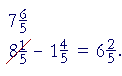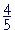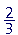S k i l l
i n
A R I T H M E T I C

Lesson 25  Section 2

SUBTRACTING MIXED NUMBERS

Back to Section 1

 Example 1. 8 45 − 1 15

Solution.  In this example, we may simply subtract the whole numbers and subtract the fractions -- similarly to adding mixed numbers.

 Example 1. 8 45 − 1 15 = 7 35 .

But consider the following, in which the fractions are reversed:

 Example 1. 8 15 − 1 45 .
 How can we deal with that?  We cannot take 45 from 15 .

To see how to deal with it, consider the following:We cannot take 40 minutes from 10 minutes -- we need more minutes. To get them, we will break off 1 of the 7 hours, and decompose it into 60 minutes.  We then regroup them with 10 minutes.

60 minutes + 10 minutes = 70 minutes:2 hours from 6 hours is 4 hours.  40 minutes from 70 minutes is 30 minutes.Solution.  We cannot take 11 inches from no inches.  To make inches, then, from 8 feet we will take 1 foot -- which is 12 inches:5 feet from 7 feet is 2 feet.  11 inches from 12 inches is 1 inch.

 8 15 − 1 45 .

We need more fifths. Where will we get them?  From 8.  We will break off 1 from 8, and decompose it into.  (Lesson 21, Example 4.)We will then add thosewith the originalmaking a total of.

Then:

 "1 from 7 is 6. 45 from 65 is 25 ."

*

Actually, the simplest way to do this problem is mentally, by rounding off 1to 2. (Compare Lesson 7.) That is, addto both numbers.

8− 1= 8− 2 = 6.Break off 1 from 4.  Express it as 88 , and add it to 18 :

Now the mystery, if any, is:  How does that numerator get to be 9?

9 is the sum of the original numerator 1 and denominator 8:Example 5.   Write the missing numerator:  9 25 = 8 ?5

7 is the sum of denominator plus numerator:  5 + 2.

 Example 6.      9 27 − 3 57 = ?9 27 becomes 8 97 .  The improper numerator 9 is the sum of the
 denominator and numerator of 27 :   7 + 2 = 9.
 Example 7. 6 − 1 23 = 5 33 − 1 23 = 4 13 .
 "1 from 5 is 4. 23 from 33 is 13 ."

Again, the simplest way to do this is mentally by rounding off 1to 2.  Add. (Lesson 21.)

The problem then becomes

6 − 1= 6− 2 = 4.

 Example 8.   Prove:   6 − 1 23 = 4 13

Solution.   According to the meaning of subtraction,

 1 23 + 4 13 = 5 33 = 6.

Compare Lesson 7, Example 2.

 Example 9. 6 12 − 2 34

Solution.  First, we must make the denominators the same:

 Example 10. 6 12 − 2 34 = 6 24 − 2 34

We cannot takefrom; therefore, on taking—1—from 6, the fraction becomes 4-fourths + 2 -fourths = 6-fourths:

 Example 9. 6 12 − 2 34 = 5 64 − 2 34 = 3 34 .
 "2 from 5 is 3. 34 from 64 is 34 ."

Mentally:

 6 12 − 2 34 . "3 from 6 12 is 3 12 Plus 14 is 3 34 ."

Please "turn" the page and do some Problems.

or

Continue on to the next Lesson.

Previous Section